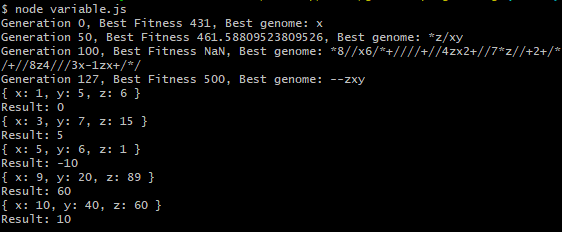# Creating Self-Assembling Code with Genetic Programming

## Introduction

Genetic programming is a branch of artificial intelligence that uses evolutionary computation to generate computer programs for solving a particular task. The computer programs are represented by encoded genes and slightly modified at each time-step via crossover and mutation until a resulting program is found that solves the given task to a degree of accuracy.

Genetic algorithms can be used for a variety of purposes, ranging from searching large datasets, tuning neural networks, and even for creating programs that write their own programs.

It can be fascinating to watch an AI write its own computer programs from scratch, which was the motivation behind the research work into self-programming AI. Although, traditionally, genetic algorithms have been used for generating software programs through the technique of genetic programming.

While the greater category of genetic algorithms involve using evolutionary techniques in software to generate a continuously better solution to a target problem, genetic programming applies this principle directly to a specific programming language. Genetic programming involves manipulating programming instructions, usually through a tree structure, crossing over branches, nodes, and mutating instructions until the resulting tree (i.e., computer program) successfully solves the task at hand. The final product of a genetic programming solution would consist of an array of instructions, possibly encoded within the genome, that correspond to programming instructions in the given language.

Care must be taken when choosing the desired programming language to use with genetic programming, due to the potential explosion of combinations of instructions, operands, operators, looping constructs, and syntax.

In this article, we’ll take a look at a simple example of implementing genetic programming for solving a mathematical formula. We’ll design a genetic program that can represent a solution for a math expression using Polish notation, specifically using prefix notation. We’ll walk through the steps of developing the genetic algorithm in JavaScript, designing the fitness function, applying the evolutionary techniques of crossover and mutation on the math expression trees, and deriving the target solution to a math formula.

## What Are We Trying to Build?

A genetic algorithm can be thought of as a search algorithm. Initially, a genetic algorithm uses a collection of randomly initialized genomes. Each genome consists of an encoded set of instructions that we can execute and determine a fitness score. The genomes are then ranked according to their fitness, the best genomes are combined together, and the next generation of genomes continues the process.

For this tutorial, we’re going to design a genetic algorithm for math formulas. We’ll be using prefix notation to represent the genomes, as this allows for the genome instructions to be easily translated from a tree structure to a readable format.

## Infix, Postfix, and Prefix Notation

Polish notation is a form of representing mathematical expressions. The type of expressions that we’re usually used to seeing are in infix format. An example of an infix expression is shown below.

By contrast, a postfix expression changes the position of the operators by moving them towards the right of the operands. An example of a postfix expression is shown below.

You can see the evaluation process for the above postfix expression is the tree shown below.Similar to the postfix expression, prefix format moves the operators to the left of the operands. An example of a prefix expression is shown below.

A convenient feature of infix, postfix, and prefix math expressions is that they can each be converted into the other expression formats. Prefix can be converted to postfix. Prefix can also be converted to infix.

The following two results are the output of two conversion programs that translate prefix to postfix and infix respectively.

Similarly, a more lengthy prefix expression is shown below. This expression evaluates to the value `123`.

`+8+*8+7-80-38`

Here is the same expression translated into postfix notation.

`88780-+*38-++`

We can evaluate the result of this expression by using the following steps shown below.

We can visualize this process in the following chart.## The Problem to Solve

Our genetic AI’s task is going to be to develop a program for a specific math expression. For example, we could ask the AI to create a program, using prefix notation, that will result in the value `6`.

Any of the example expressions above would suffice as a solution to this task. Since we’re using prefix notation, specifically, the last example would serve as a solution. For example, any of the following results could be used as a solution for a program that calculates the value `6`.

It’s up to the genetic algorithm, and its associated fitness function, to derive the resulting program. By adjusting various features of the fitness function to score different traits and qualities of the programs, the final resulting program can be targeted for shortest length, least instructions, largest or smallest values, etc.

## A Little More Interesting

We will develop a genetic algorithm for creating a program to output a discrete value. However, to make our program a little more interesting and impressive, we’ll have our genetic algorithm also create a solution math expression for a formula containing variables.

Suppose that we have several samples of data, such as, `(X=1, Y=2)`, `(X=2, Y=3)`, `(X=3, Y=4)`. We want the genetic algorithm to come up with the math formula that represents the data above. In this case, the formula `Y = X + 1` is a suitable solution. Similarly, so is `Y = 1 + X * 1`. There are a variety of potential solution, but coming up with the result is the key. Further, the resulting program’s math formula should generalize and be correct for any value of X.

This can be particularly interesting for series of data where you may not be sure what the originating math expression is. Consider complex sets of variables with just a handful of sample data points. These could be supplied to the genetic algorithm and a resulting math formula output as the solution to satisfy those data points. If the sample size is sufficient enough, you could discover a brand new math formula for calculating the result of any combination of your variables.

Now that we have a basic understanding of Polish notation format for representing math expressions, we need to design a way to use them with a genetic algorithm.

## Genetic Algorithms in JavaScript

The genetic-js library facilitates the construction of a genetic algorithm in JavaScript. It provides the necessary functions and handlers for defining a genetic algorithm, its initialization, a fitness function, and a means for ranking solutions. In this manner, a genetic algorithm can be developed for a large variety of tasks, and as shown in this project, genetic programming.

## Initialization

Usage of the genetic algorithm library in node.js is relatively straight-forward. You can begin by instantiating the genetic class and selecting an optimization method and selection algorithm.

In the above example, we’ve instantiated the genetic class and selected to maximize the fitness among the pool of genomes. The best performing results will continue on to the next epoch of evolution.

## Creating the Initial Population

After creating the initial genetic algorithm class, you can provide a function of creating the initial pool of genomes to begin evolution from. This set of genomes is usually randomly produced, with some of the genomes performing better than others for solving the desired task. It’s these better performing genomes that will rank higher and move on to the next epoch of evolution, creating the next generation.

## Crossover

When creating the next population of genomes after an epoch, the methods of crossover and mutation are used to slightly modify the existing genomes and create children. Crossover is the process of creating a child genome by taking two parent genomes and mating them together to produce offspring. This is done by taking a series of genes from parent A and parent B and combining them together to form a child. Additional mutation can be used on the child to slightly tweak the genes further, which has the potential to produce beneficial (or worse) functionality for the child.

A simplified example for performing crossover on two parent genomes to produce two child genomes is shown below.

In the above code, we’re simply splitting the two parent genomes at a specific index. We then take the left portion of one parent and combine it with the right portion of the other parent to produce a child offspring. We repeat this a second time for the second child offspring, by taking the right portion of the first parent and combine it with the left portion of the second parent.

## Mutation

After performing crossover, each child genome can be slightly modified a bit further by mutating specific genes. This may or may not produce beneficial effects that can boost the child’s resulting fitness score. Mutation can be performed by randomly altering the value of a gene or by inserting and removing genes at specific indices within the genome.

In the above code, we’re performing mutation by replacing a randomly selected single gene within the child. We modify the gene by either incrementing or decrementing it by a value of 1. In the case of letters representing the genes, this would select either the previous or next letter within the alphabet. For numbers, this may add or subtract some fraction from the original value.

## Fitness

Scoring the fitness for a genome is the most important task of developing a genetic algorithm, as this contains the core logic for guiding which genomes are to be selected for producing the next generation of genomes and thus, solving a particular task. A granular method of scoring the fitness for each genome is required in order to allow the genetic algorithm to rank genomes accordingly, even if they don’t solve the entire given task. By assigning partial credit to those genomes which perform just slightly better than others, the evolution process can select better performing programs in a granular fashion until it arrives at a solution.

In the above example, we’re simply scoring the fitness according to how close each letter within the genome is to the target letter. For example, to output the string “Hello World”, if the first letter in genome 1 is a ‘G’ and the first letter in genome 2 is a ‘T’, then the first genome will be ranked higher by fitness, since ‘G’ is closer to ‘H’ (the first target letter) than ‘T’. Of course, the entire score for the genome is taken into account. If the second genome had a worse score for the first gene (‘T’), but had all of its other genes (i.e., letters) closer to the solution, then it would end up with a higher scoring fitness and thus move on to the next generation.

## Termination

Ending the selection process for a genetic algorithm can be done by specifying a target fitness score or a target solution. This can be done by simply comparing the fitness of the highest ranking genome against the expected target solution.

In the above example, we’re taking the highest ranking genome `pop` and checking its fitness score. For the case of outputting the string “Hello World”, if all of its characters are correct for the target string, then its fitness will be a perfect score of `256 * solution.length`, or a score of `256` for each letter in the string.

## Displaying Status While Evolving

It can be helpful to view the output during the evolution process. This can be done by implementing the notification method as shown below.

In the above example, we’re simply outputting the generation number along with the best performing genome (which in the case, is encoded as a set of letters - i.e., the text that we’re trying to generate).

## Running the Genetic Algorithm

To run the genetic algorithm and start the evolutionary process, we simply call the `evolve` method. A number of configuration parameters can be provided, including the maximum iterations, population size, crossover rate percentage, and mutation rate percentage. We can also store data for use by our methods (such as the target string to be generated, etc.).

## Hello World

The traditional “hello world” of genetic algorithms is to have the AI develop a program for outputting the string. We can do this with the genetic-js library using the code snippets above. The AI starts with a completely random string of characters for each genome. It must use the evolutionary techniques of crossover and mutation to gradually change the genomes into the target string.

The key piece of the genetic algorithm is the fitness function. In the case of hello world, the fitness function determines the character distance from each letter in the generated program’s output to the target string. The closer the letters get to the target fitness, the higher their score will be. In this manner, we can guide the AI to get better and better with the generation process until the correct target string is achieved.In the above screenshot, notice how the genome for the target string “Hello World” is gradually adjusted at each generation until the complete solution is achieved at generation 248.

This same technique can be used for evolving math expressions too!

## Solving a Discrete Math Expression

Now that we’ve demonstrated how the genetic algorithm framework works within JavaScript to output a simple string of “Hello World”, we can modify the initialization and fitness methods to instruct the AI to generate a different solution.

So far, the genetic algorithm has generated a string. In our example case, this was the string “Hello World”. We designed the fitness method to sum the difference between each character generated from the target string, until the generated characters matched exactly, arriving at our solution.

This time, we’re going to generate programming code. The code that is generated will actually be prefix expression notation, and will consist of operands (i.e., the digits 0-9) and operators (i.e., + - * /). The entire genome will represent a prefix expression that can be evaluated to calculate a resulting numeric answer. Our fitness method will score the genome according to how close the calculated result from the prefix expression is to our target value.

## Generate a Program to Calculate 123

As a first example for generating a discrete math formula, let’s try to generate a program that calculates the value `123`.

Since we’re using prefix notation format, we’ll first need to randomly initialize the genomes to contain the set of programming instructions (valid operands and operators). Additionally, since we know that we’re generated prefix expressions, we can take advantage of the tree structure of Polish notation format to generate valid expression trees.

## Generating a Random Prefix Expression Tree

A valid expression tree will consist of nodes as operands or operators. We can generate a random tree by selecting a random operand or operator as the starting node. If the node is an operand (i.e., a numeric value) we stop and the tree is complete. If the node is an operator (+ - * /), we repeat the process to generate two child nodes for the parent. The child nodes, in turn, may be operands and operators, randomly generating a tree until all final leaf nodes consist of operands. It’s also a good idea to cut off the generation process at a maximum depth, to avoid overly deep expression trees. In this case, after a certain depth, any generation of nodes can be strictly set to operands only, which avoid generating further child operator/operand combinations.

Below is an example of randomly generating a prefix expression tree.

Notice in the above code how we recursively call the `tree()` method to create left and right child branches while randomly generating a tree. If a node is an operator, we continue recursively generating child branches. Otherwise, if the node is an operand, we simply return it and end the leaf node.

In addition to generating an expression tree, we’ll need a way to evaluate it to calculate the resulting value.

## Evaluating Expression Trees

A postfix expression tree can be evaluated with an algorithm that simply walks the array of instructions from left to right. Likewise, a prefix expression tree can be evaluated by walking in the opposite direction, right to left.

Let’s briefly take a look at the algorithm and code for evaluating a postfix and prefix expression tree, as these will be used for calculating the fitness for each genome.

## Evaluating a Postfix Expression Tree

Evaluating a postfix expression tree can be done by traversing the array of instructions (operands and operators) and applying them in order from left to right. The code for evaluating a postfix expression is shown below.

The above code evaluates a postfix expression tree. The code begins by evaluating from left to right. If the instruction is a value, we simply push it on the stack. If the value is an operator, we pop two items from the stack and apply the operator to obtain a resulting value. We then push that value back on the stack.

Keep in mind, when evaluating a postfix expression, the operator comes after the operands. Due to this, the first operand from the stack will be the right-hand operand and the second will be the left-hand one.

As an example, consider the string `5 3 -`. The stack will contain `[5, 3]` with `3` being at the top of the stack. The operator is a `-`. Therefore, the first `pop()` gives us `3` which is the right-hand operand. The second `pop()` gives us `5`. We can then calculate `5 - 3 = 2`. If the order is reversed, the resulting value would be `3 - 5 = -2` which is not correct. This is the reason that we first pop the value into the variable `b` and the second into the variable `a` to perform `a op b`.

## Evaluating a Prefix Expression Tree

Similar to evaluating a postfix expression tree, we can evaluate a prefix expression tree by reversing the direction of traversal. Instead of reading from left to right, we’ll start at the end of the array and walk backwards to the beginning. The code for evaluating a prefix expression is shown below.

Notice in the above code, we start from the end of the array and begin working towards the front. We follow the same algorithm for pushing operands onto the stack and popping twice from the stack when an operator is found.

Recall, when evaluating a postfix expression tree we had to reverse the order of the operands as obtained from the stack, in order to perform the calculation. However, this time when an operator is encountered, the operands will already be in the correct order (since we’re walking backwards), thus we can read them in-order and perform the calculation for `a op b`.

Let’s use the same example from the postfix expression tree evaluation as an example.

Consider the string `- 5 3`. Since we walk backwards (starting from the end of the string) for prefix evaluation, the stack will contain `[3, 5]` with `5` being at the top of the stack. This is reversed from what we saw when performing postfix evaluation. The operator is a `-`. Therefore, the first `pop()` gives us `5` which is the left-hand operand. The second `pop()` gives us `3`. We can then calculate `5 - 3 = 2`, without the need to swap the order of the operands as we had to do with postfix evaluation.

## Calculating the Fitness for an Expression Tree Genome

The fitness method is the core part of a genetic algorithm. In the case of evaluating the fitness for an expression tree, we can subtract the absolute difference of the target value and the actual value from the tree from the target value. This effectively makes the target value the top fitness score to achieve. Any deviation from that value applies a penalty to the fitness score.

The code example below demonstrates this.

For example, consider the case where we’re trying to have the genetic algorithm develop a program that results in the value 10. If an expression tree evaluates to a value of 8, then the fitness for this genome is `10 - abs(10 - 8) = 8`. This is pretty close to the target solution. Likewise, if a different expression tree evaluates to a value of 25, then the fitness for this genome is `10 - abs(10 - 25) = -5`. The second tree has a lower fitness score than the first, as it’s value is further from the target. As one final example, an expression tree that evaluates to a value of 9 would have the same fitness score of `10 - abs(10 - 9) = 9` as a tree that evaluates to a value of 11 with `10 - abs(10 - 11) = 9`. Both trees in this case are the same distance from the target and thus rank the same according to fitness.

## Crossover for Expression Trees

After ranking the genomes according to fitness, pairs of parent genomes can be selected for mating. This is typically done with a roulette style of selection, where higher scoring genomes have a higher percentage likelihood of reproducing, but not 100%. If you use the technique of elitism, the highest scoring genomes will always carry forward (although this can have the side-effect of producing a local maximum or less than optimal solution).

Once two parent genomes are selecting for producing children, we enact two processes to generate the new genomes. The first process involves crossover.

Crossover takes a portion of the genes from the first parent and combines it with a portion of the genes from the second. The resulting genome has the same length as the parent genomes and shares a number of its genes with each parent.

The decision factor is the number of genes to take from each parent. This is usually decided via a random factor, which may take the first 5 genes from parent 1 and the last 5 genes from parent 2 (if generating a genome of length 10).

In the case of expression trees, we’re actually copying a sub-tree from parent 1 and appending it onto the child along with a sub-tree from parent 2. However, since we’re cutting sub-trees, they will need to be valid executing trees on their own. That is, we can’t simply cut a sub-tree at an operand and append it to the child tree at a location that already has two operands connecting to an operator (operators, in our case, alway takes two child operands).

A valid sub-tree can be extracted from an expression tree by following the same logic as the evaluation process for prefix or postfix notation. The difference is that we stop evaluating once a specific index level is reached. Once we reach the desired index within the tree, we can extract the string of values that represent the tree nodes from the starting index to the ending sub-tree nodes.

The code for performing a crossover of expression tree genomes can be found below.

Notice in the above code, we take two parent genomes and create two child genomes. We do this by first selecting two random indices. We then extract the subtrees from the parent at the random indices. Finally, we crossover the subtrees between the children to produce the resulting genomes.

## Mutating an Expression Tree

After creating a child genome via the crossover process from two parent genomes, we can add an additional factor of diversity by randomly mutating a small number of genes.

Mutation changes specific genes of a genome by a designated value, thus altering the behavior of the result program. The resulting expression tree may achieve a higher or lower fitness score, depending on the type of mutation enacted.

In the case of expression trees, we need to ensure the trees remain as valid. To do this, we can restrict the mutation of genes to follow the same type (operand or operator) as the originating gene. That is, if an operator gene is selected for mutation, we replace it with a different operator. Likewise, if an operand gene is selected for mutation, we replace it with a different operand.

The code for performing mutation on an expression tree can be found below.

Notice in the above code that we select a random index within the genome to mutate. If this gene is currently an operator, we mutate by replacing it with a random selection from the list of available operators. Likewise, if the gene is currently an operand, we mutate by replacing it with a random selection from the list of available operands (i.e., a new value).

There are other techniques of crossover and mutation that can be performed to optimize performance. The above represent a convenient starting point for a genetic algorithm.

## Results

### Hello World

Earlier, we described the genetic algorithm code for implementing a classic “hello world” example. The goal of the genetic algorithm was to evolve a random set of characters into the complete sentence, “Hello World”. The AI generated this solution in 248 generations. An example of the result of this process is shown below### Prefix Expression Tree for the Value 123

The results of generating a program that evaluates to the value `123` is shown below for two separate runs of the genetic algorithm, thus producing two different solutions for expression trees that evaluate to `123`.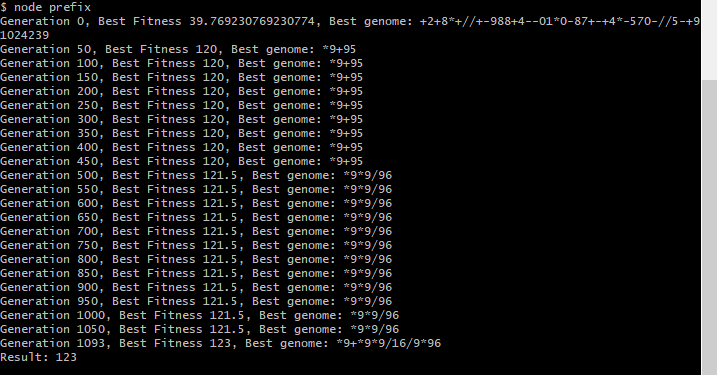The above example shows an initial run of the genetic programming solution to generate a program to output the value `123`. The genetic algorithm includes no limit on the depth of the generated expression tree.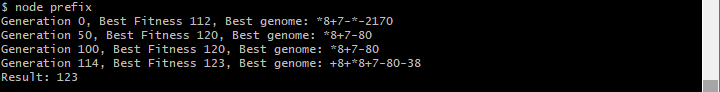A second run of the genetic algorithm, as shown above, achieved a result in a much shorter duration of time. This was due to restricting the length of generated expression trees.

### Prefix Expression Tree for the Value 123456

Similar to the above example, we can generate programs to evaluate larger numbers, such as `123456`. Naturally, the resulting expression tree will be longer and more complex in order to achieve the target value.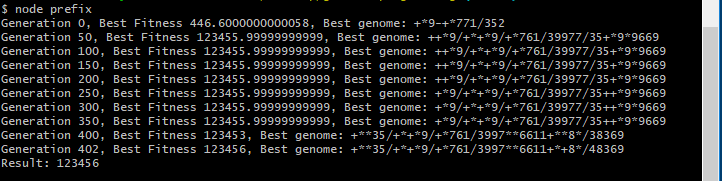You can see in the result of the genetic algorithm that further optimization could be used. For example restricting the length of the expression tree or favoring shorter results (i.e., number of instructions) when calculating the fitness score.

## Solving Calculations Containing Variables

So far, we’ve demonstrated using genetic programming to generate programs that evaluate to a discrete value. More interesting, however, is to have the AI generate a program for a more complex mathematical function containing variables and unknowns. In this manner, we could provide the genetic algorithm with several training data examples of, for example, X and Y values. We could then ask the AI to generate the math formula that corresponds to these X and Y values. The goal would be a resulting program that could accurately calculate the result for Y for any given value of X.

The power of genetic programming and AI in this case is that by only providing a limited number of training examples, the AI could still generate a solution that generalizes from the training data to produce a resulting program that holds valid for new values of X and Y that have never been seen before. This is particularly powerful for cases where a calculation may be complex or simply have too many unknowns, or perhaps not enough data samples. In these cases, the known training examples can be provided and the genetic algorithm can attempt to locate a solution that holds valid for all training cases - including new data!

### Writing a Program to Solve the Third Power

Let’s take an example of having the AI write a program that calculates X^3. That is, for any value of X that you provide to the program, the prefix expression tree will evaluate X*X*X.

An initial run of the program produces the following output shown below.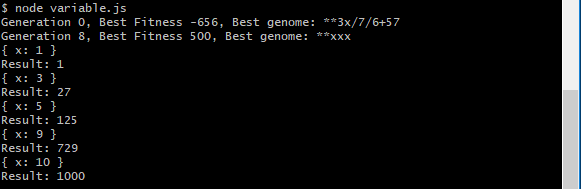Notice, we’ve provided five test cases to the genetic programming algorithm to base its generation on. If it can write an expression that correctly applies to all test cases, we can hope that the resulting program generalizes correctly for any value of X. In the above result, a program was generated of `**xxx`. This will indeed generalize for any value of X to produce X^3 and is thus a correct result.

A second run of the generation produces the following expression as shown below.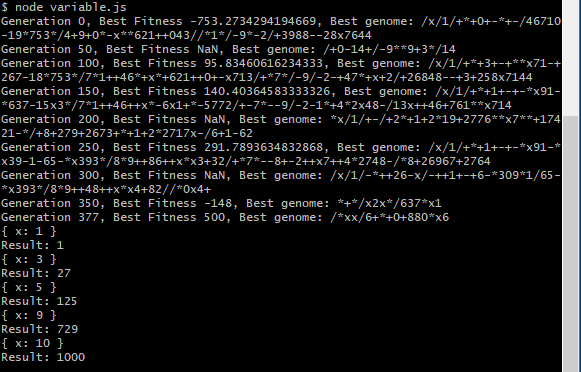The output generated the prefix expression `/*xx/6+*+0+880*x6`. This program correctly solves the provided test-cases, as shown in the output. However, does it generalize? It’s difficult to tell by simply looking at the generated program. Let’s try running the resulting program on several different values for X.

First, we can translate the prefix expression into its postfix version and obtain the result of `xx*6088++0*x6*+//`.

Let’s evaluate the steps for this expression for a value of `X = 2`, so `X^3 = 8`.

While the expression tree uses a complicated means of reaching X^3, this is indeed a correct result. Let’s try it on one more number for `X = 7`. The steps are shown below.

Indeed, the above steps result in a fractional, but correct answer of `342.9999999` which rounds to `X^3 = 7*7*7 = 343`. You can also try this with other values, such as `35^3`, and find that the result correctly evaluates to `42875`.

### Writing a Program to Solve Z - (X + Y)

Programs can be generated for more complex expressions as well, such as ones containing multiple variables. The result from generating a program to solve `Z - (X + Y)` produces the result of `--zxy`. In postfix, this is translated to `zx-y-`. In infix, this is translated to `(z-x)-y`. We can confirm this is a correct result since both `z-(x+y)` and `(z-x)-y` simplify to `-x-y+z`.

The result is displayed below.